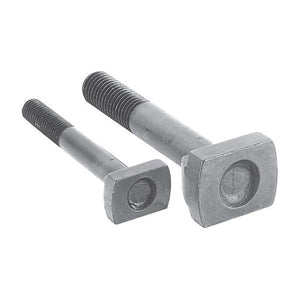## Categories# T-Slot bolts

• DIN 787, Tensile Strength 8,8
• Heat treated alloy steel.¬†Ôªø
• Black oxide finish
• Completely finished machined head provides 50% more bearing surface
• Longer life than standard hot-forged type
M12 1/2" 90 A=22.2, B=26.9, C=15.2, E=12.7, F=7.1, G=4.6 TS1290 9.95 - +
M12 1/2" 100 A=22.2, B=26.9, C=15.2, E=12.7, F=7.1, G=4.6 TS12100 10.15 - +
M12 1/2" 160 A=22.2, B=26.9, C=15.2, E=12.7, F=7.1, G=4.6 TS12160 11.20 - +
M16 16mm 100 A=26.9, B=31.8, C=19.8, E=15.7, F=9.4, G=6.4 TS16100 10.50 - +
M16 16mm 125 A=26.9, B=31.8, C=19.8, E=15.7, F=9.4, G=6.4 TS16125 12.00 - +
M16 16mm 150 A=26.9, B=31.8, C=19.8, E=15.7, F=9.4, G=6.4 TS16150 12.50 - +
M16 16mm 180 A=26.9, B=31.8, C=19.8, E=15.7, F=9.4, G=6.4 TS16180 14.50 - +
M16 16mm 200 A=26.9, B=31.8, C=19.8, E=15.7, F=9.4, G=6.4 TS16200 16.25 - +
M20 20mm 100 A=34, B=40, C=28, E=20, F=14, G=10 TS20100 12.22 - +
M20 20mm 120 A=34, B=40, C=28, E=20, F=14, G=10 TS20120 12.88 - +
M20 20mm 150 A=34, B=40, C=28, E=20, F=14, G=10 TS20150 17.01 - +
M20 20mm 200 A=34, B=40, C=28, E=20, F=14, G=10 TS20200 17.43 - +
M24 24mm 150 A=42, B=48, C=34, E=24, F=16, G=10 TS24150 26.08 - +
M24 24mm 200 A=42, B=48, C=34, E=24, F=16, G=10 TS24200 27.04 - +